# Specific requirements

In this programming project, complete the following steps:
• Select a data set and load it into R.
• Apply time series regression models to analyze the data of your own choosing.
• Submit a *.html file generated by R Markdown.

# Data frame information

Title:
Weekly stock data for Dow Jones Index
Source:
This dataset comprises data reported by the major stock exchanges.
Past Usage:
This dataset was first used in:

Brown, M. S., Pelosi, M. & Dirska, H. (2013). Dynamic-radius Species-conserving Genetic Algorithm for the Financial Forecasting of Dow Jones Index Stocks. Machine Learning and Data Mining in Pattern Recognition, 7988, 27-41.

We request that you provide a citation to this paper when using the dataset. We welcome you to compare your results against ours in (Brown, Pelosi & Dirska, 2013).

Relevant Information:
In predicting stock prices you collect data over some period of time - day, week, month, etc. But you cannot take advantage of data from a time period until the next increment of the time period. For example, assume you collect data daily. When Monday is over you have all of the data for that day. However you can invest on Monday, because you don’t get the data until the end of the day. You can use the data from Monday to invest on Tuesday.

``````In our research each record (row) is data for a week.  Each record also has the percentage
of return that stock has in the following week (percent_change_next_weeks_price). Ideally,
you want to determine which stock will produce the greatest rate of return in the following

Some of these attributes might not be use used in your research.  They were
originally added to our database to perform calculations.  (Brown, Pelosi & Dirska, 2013)
used percent_change_price, percent_change_volume_over_last_wk, days_to_next_dividend,
and percent_return_next_dividend.  We left the other attributes in the dataset
in case you wanted to use any of them. Of course what you want to maximize is
percent_change_next_weeks_price.

Training data vs Test data:
In (Brown, Pelosi & Dirska, 2013) we used quarter 1 (Jan-Mar) data for training and
quarter 2 (Apr-Jun) data for testing.

Interesting data points:
If you use quarter 2 data for testing, you will notice something interesting in
the week ending 5/27/2011 every Dow Jones Index stock lost money.

The Dow Jones Index stocks change over time.  The stocks that made up the index in 2011 were:
3M:MMM
American Express:AXP
Alcoa:AA
AT&T:T
Bank of America:BAC
Boeing:BA
Caterpillar:CAT
Chevron:CVX
Cisco Systems:CSCO
Coca-Cola:KO
DuPont:DD
ExxonMobil:XOM
General Electric:GE
Hewlett-Packard:HPQ
The Home Depot:HD
Intel:INTC
IBM:IBM
Johnson & Johnson:JNJ
JPMorgan Chase:JPM
Kraft:KRFT
McDonald's:MCD
Merck:MRK
Microsoft:MSFT
Pfizer:PFE
Procter & Gamble:PG
Travelers:TRV
United Technologies:UTX
Verizon:VZ
Wal-Mart:WMT
Walt Disney:DIS
``````

Number of Instances:
There are 750 data records. 360 are from the first quarter of the year (Jan to Mar).

390 are from the second quarter of the year (Apr to Jun).

Number of Attributes:
There are 16 attributes.

For each Attribute:

``````quarter:  the yearly quarter (1 = Jan-Mar; 2 = Apr=Jun).
stock: the stock symbol (see above)
date: the last business day of the work (this is typically a Friday)
open: the price of the stock at the beginning of the week
high: the highest price of the stock during the week
low: the lowest price of the stock during the week
close: the price of the stock at the end of the week
volume: the number of shares of stock that traded hands in the week
percent_change_price: the percentage change in price throughout the week
percent_chagne_volume_over_last_wek: the percentage change in the number of shares of stock that traded hands for this week compared to the previous week
previous_weeks_volume: the number of shares of stock that traded hands in the previous week
next_weeks_open: the opening price of the stock in the following week
next_weeks_close: the closing price of the stock in the following week
percent_change_next_weeks_price: the percentage change in price of the stock in the following week
days_to_next_dividend: the number of days until the next dividend
percent_return_next_dividend: the percentage of return on the next dividend``````

Missing Attribute Values:
None

Data Reference:
http://archive.ics.uci.edu/ml/datasets/Dow+Jones+Index

# Include the knitr package for integration of R code into Markdown

``knitr::opts_chunk\$set(echo = TRUE)``

# Import data

``````library(readr)
input_data <- read_csv("dow_jones_index.csv", col_types = cols(

stock = col_character(),
date = col_date(format = "%m/%d/%Y"),
open = col_number(),
close = col_number(),
volume = col_number(),

days_to_next_dividend = col_skip(),
high =  col_skip(),
low =  col_skip(),
next_weeks_close =  col_skip(),
next_weeks_open =  col_skip(),
percent_change_next_weeks_price = col_skip(),
percent_change_price =  col_skip(),
percent_change_volume_over_last_wk = col_skip(),
percent_return_next_dividend = col_skip(),
previous_weeks_volume = col_skip(),
quarter = col_skip()

))``````

# Descriptive statistics

## Dimension of data frame

``dim(input_data)``
``##  750   5``

## Structure of data frame

``str(input_data)``
``````## tibble [750 x 5] (S3: spec_tbl_df/tbl_df/tbl/data.frame)
##  \$ stock : chr [1:750] "AA" "AA" "AA" "AA" ...
##  \$ date  : Date[1:750], format: "2011-01-07" "2011-01-14" ...
##  \$ open  : num [1:750] 15.8 16.7 16.2 15.9 16.2 ...
##  \$ close : num [1:750] 16.4 16 15.8 16.1 17.1 ...
##  \$ volume: num [1:750] 2.40e+08 2.43e+08 1.38e+08 1.51e+08 1.54e+08 ...
##  - attr(*, "spec")=
##   .. cols(
##   ..   quarter = col_skip(),
##   ..   stock = col_character(),
##   ..   date = col_date(format = "%m/%d/%Y"),
##   ..   open = col_number(),
##   ..   high = col_skip(),
##   ..   low = col_skip(),
##   ..   close = col_number(),
##   ..   volume = col_number(),
##   ..   percent_change_price = col_skip(),
##   ..   percent_change_volume_over_last_wk = col_skip(),
##   ..   previous_weeks_volume = col_skip(),
##   ..   next_weeks_open = col_skip(),
##   ..   next_weeks_close = col_skip(),
##   ..   percent_change_next_weeks_price = col_skip(),
##   ..   days_to_next_dividend = col_skip(),
##   ..   percent_return_next_dividend = col_skip()
##   .. )``````

## Summary statistics of data frame

``summary(input_data)``
``````##     stock                date                 open            close
##  Length:750         Min.   :2011-01-07   Min.   : 10.59   Min.   : 10.52
##  Class :character   1st Qu.:2011-02-18   1st Qu.: 29.83   1st Qu.: 30.36
##  Mode  :character   Median :2011-04-01   Median : 45.97   Median : 45.93
##                     Mean   :2011-03-31   Mean   : 53.65   Mean   : 53.73
##                     3rd Qu.:2011-05-13   3rd Qu.: 72.72   3rd Qu.: 72.67
##                     Max.   :2011-06-24   Max.   :172.11   Max.   :170.58
##      volume
##  Min.   :9.719e+06
##  1st Qu.:3.087e+07
##  Median :5.306e+07
##  Mean   :1.175e+08
##  3rd Qu.:1.327e+08
##  Max.   :1.453e+09``````

``head(input_data)``
``````## # A tibble: 6 x 5
##   stock date        open close    volume
##   <chr> <date>     <dbl> <dbl>     <dbl>
## 1 AA    2011-01-07  15.8  16.4 239655616
## 2 AA    2011-01-14  16.7  16.0 242963398
## 3 AA    2011-01-21  16.2  15.8 138428495
## 4 AA    2011-01-28  15.9  16.1 151379173
## 5 AA    2011-02-04  16.2  17.1 154387761
## 6 AA    2011-02-11  17.3  17.4 114691279``````

## Tail of data frame

``tail(input_data)``
``````## # A tibble: 6 x 5
##   stock date        open close    volume
##   <chr> <date>     <dbl> <dbl>     <dbl>
## 1 XOM   2011-05-20  80.2  81.6  86758820
## 2 XOM   2011-05-27  80.2  82.6  68230855
## 3 XOM   2011-06-03  83.3  81.2  78616295
## 4 XOM   2011-06-10  80.9  79.8  92380844
## 5 XOM   2011-06-17  80    79.0 100521400
## 6 XOM   2011-06-24  78.6  76.8 118679791``````

## Variable data types

``sapply(input_data,mode)``
``````##       stock        date        open       close      volume
## "character"   "numeric"   "numeric"   "numeric"   "numeric"``````

## Mean of each variable

``lapply(input_data[,c(2,3,4,5)],mean)``
``````## \$date
##  "2011-03-31"
##
## \$open
##  53.65184
##
## \$close
##  53.72927
##
## \$volume
##  117547801``````

## Median of each variable

``lapply(input_data[,c(2,3,4,5)],median)``
``````## \$date
##  "2011-04-01"
##
## \$open
##  45.97
##
## \$close
##  45.93
##
## \$volume
##  53060885``````

## Mode of each variable

``require(modeest)``
``## Loading required package: modeest``
``lapply(input_data[,c(3,4)],mfv)``
``````## \$open
##  37.26 44.75
##
## \$close
##  33.07 36.00 41.52 46.25``````
``# Variable \$Income has no mode. Each value repeats only once.``

## Minimum value of each variable

``lapply(input_data[,c(2,3,4,5)],min)``
``````## \$date
##  "2011-01-07"
##
## \$open
##  10.59
##
## \$close
##  10.52
##
## \$volume
##  9718851``````

## Maximum value of each variable

``lapply(input_data[,c(2,3,4,5)],max)``
``````## \$date
##  "2011-06-24"
##
## \$open
##  172.11
##
## \$close
##  170.58
##
## \$volume
##  1453438639``````

## Range of each variable

``lapply(input_data[,c(2,3,4,5)],range)``
``````## \$date
##  "2011-01-07" "2011-06-24"
##
## \$open
##   10.59 172.11
##
## \$close
##   10.52 170.58
##
## \$volume
##     9718851 1453438639``````

## Variance of each variable

``lapply(input_data[,c(2,3,4,5)],var)``
``````## \$date
##  2549.758
##
## \$open
##  1065.295
##
## \$close
##  1075.105
##
## \$volume
##  2.510263e+16``````

## Standard deviation of each variable

``lapply(input_data[,c(2,3,4,5)],sd)``
``````## \$date
##  50.49513
##
## \$open
##  32.63885
##
## \$close
##  32.78879
##
## \$volume
##  158438089``````

## Median absolute deviation of each variable

``lapply(input_data[,c(2,3,4,5)],mad)``
``````## \$date
## Time difference of 62.2692 days
##
## \$open
##  30.14126
##
## \$close
##  30.14867
##
## \$volume
##  44282986``````

# Scaling numeric variables

### Reason for scaling numeric variables:

It is easier to fit smaller numbers onto the axes. The numeric variable volume is normalized between the values of 10 and 1000.

Function for normalizing data values
Normalization = a + ( (x - Min(x) )(b - a))/( Max(x) - Min(x) )
x = variable subjected to normalization
a = lower bound = 10
b = upper bound = 1000

``````nmlz <- function(x) (10 + ((x - min(x))*(1000-10)) / (max(x)-min(x)))
input_data[,5] = nmlz (input_data[,5] )
``````## # A tibble: 25 x 5
##    stock date        open close volume
##    <chr> <date>     <dbl> <dbl>  <dbl>
##  1 AA    2011-01-07  15.8  16.4  168.
##  2 AA    2011-01-14  16.7  16.0  170.
##  3 AA    2011-01-21  16.2  15.8   98.3
##  4 AA    2011-01-28  15.9  16.1  107.
##  5 AA    2011-02-04  16.2  17.1  109.
##  6 AA    2011-02-11  17.3  17.4   82.0
##  7 AA    2011-02-18  17.4  17.3   58.2
##  8 AA    2011-02-25  17.0  16.7   94.5
##  9 AA    2011-03-04  16.8  16.6   78.4
## 10 AA    2011-03-11  16.6  16.0   81.7
## # ... with 15 more rows``````

# Plots and Graphs

## Box plots

### Explanation:

These box plots reveal the mean value, minimum value, and maximum value of each variable.

### Observation:

It appears that mean value, minimum value, and maximum value of the stock open prices are very much the same as the stock close prices. The stocks have the highest mean volumes of about 170 and lowest of about 10 (normalized values).

``````library(ggplot2)
ggplot(data = input_data, aes(x=date, y=open, fill=stock)) + geom_boxplot(outlier.colour = "red") + xlab("Date") + ylab("Open Price") + ggtitle("Box Plot of Stock Open Price")``````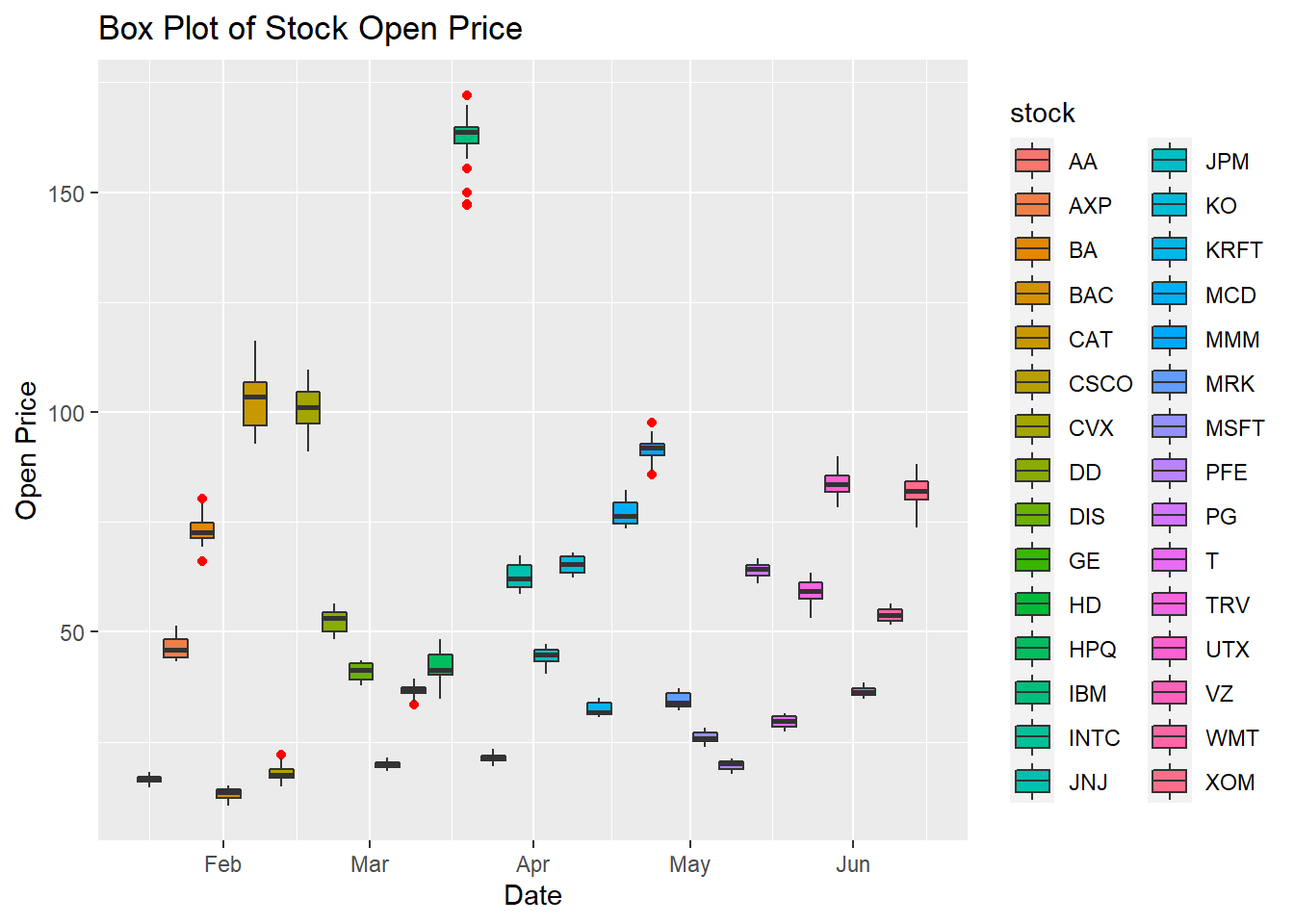``````library(ggplot2)
ggplot(data = input_data, aes(x=date, y=close, fill=stock)) + geom_boxplot(outlier.colour = "red") + xlab("Date") + ylab("Close Price") + ggtitle("Box Plot of Stock Close Price")``````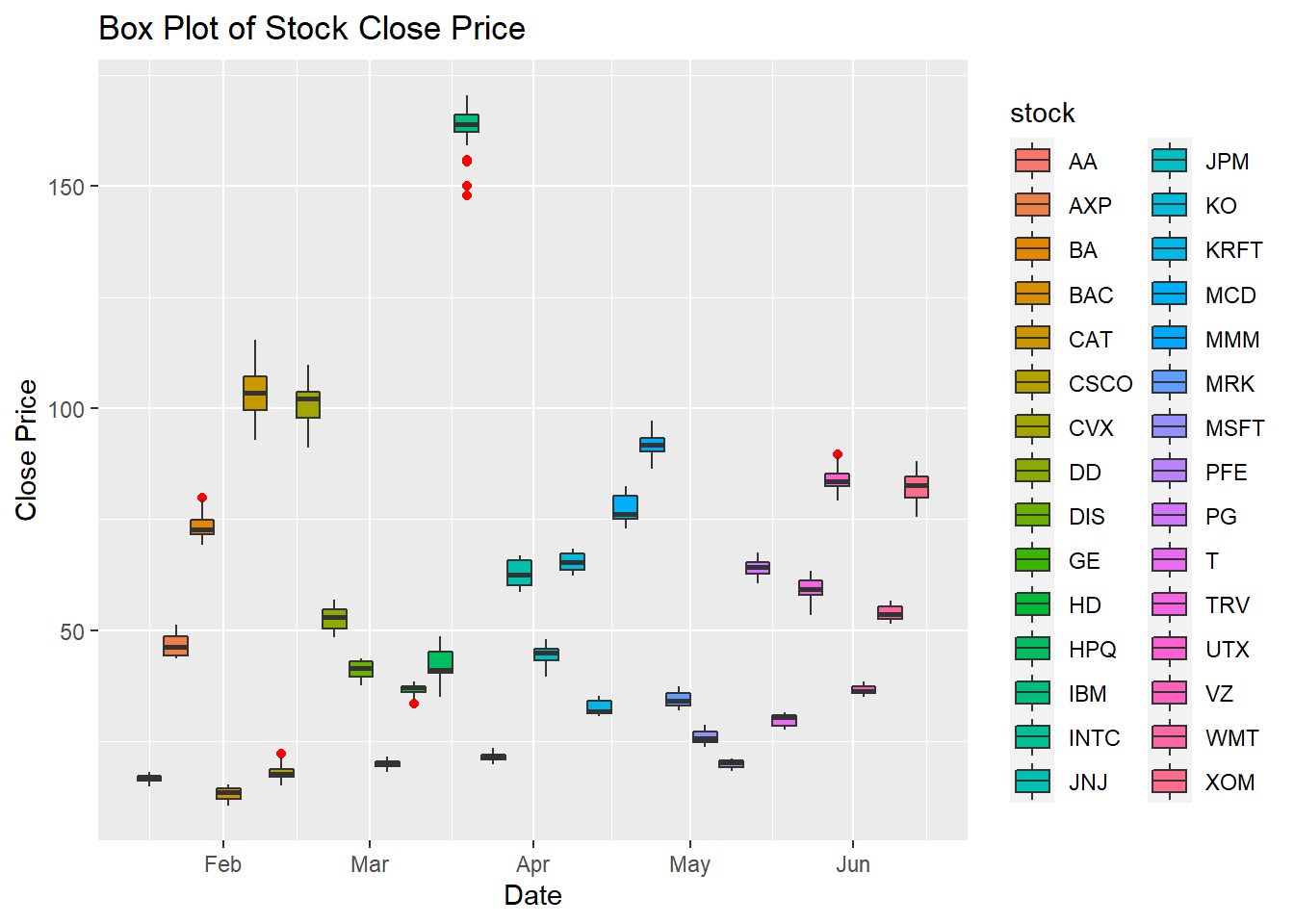``````library(ggplot2)
ggplot(data = input_data, aes(x=date, y=volume, fill=stock)) + geom_boxplot(outlier.colour = "red") + xlab("Date") + ylab("Volume") + ggtitle("Box Plot of Stock Volume")``````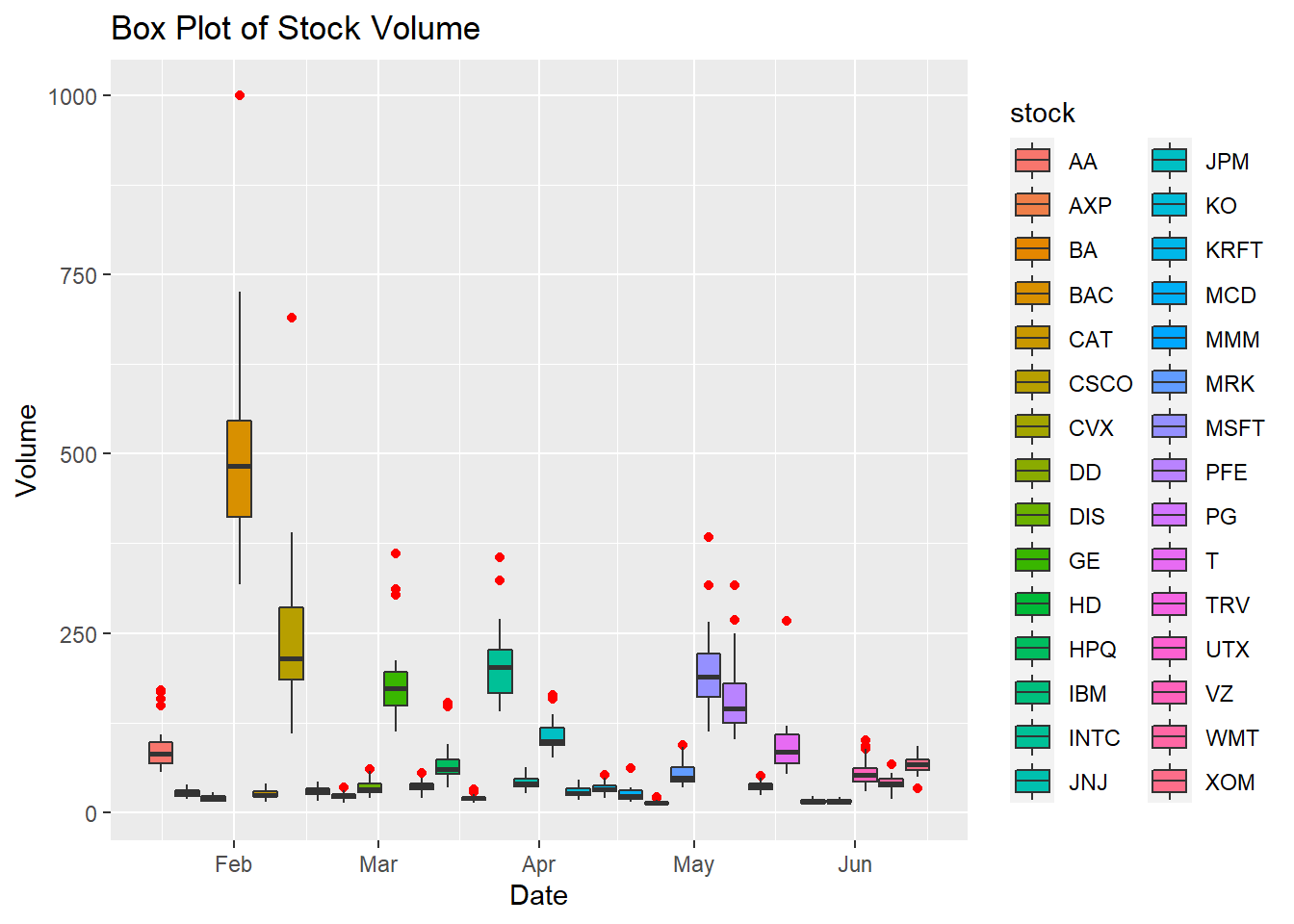## Scatter plots

### Explanation:

These scatter plots reveal the relationships between the variables.

### Observation:

It is appearance that there is a very strong positive linear relationship between the stock open price and the stock close price. Therefore, Generalized Linear Model is the appropriate time series model for this data. It is appearance that there is a negative relationship between the stock close price and the stock volume. It is appearance that out of the 30 stocks, IBM has the highest stock price.

``````library(ggplot2)
ggplot(data = input_data, aes(x=open, y=close, color=stock)) + geom_point(size=1) + xlab("Open Price") + ylab("Close Price") + ggtitle("Close Price Vs. Open Price")``````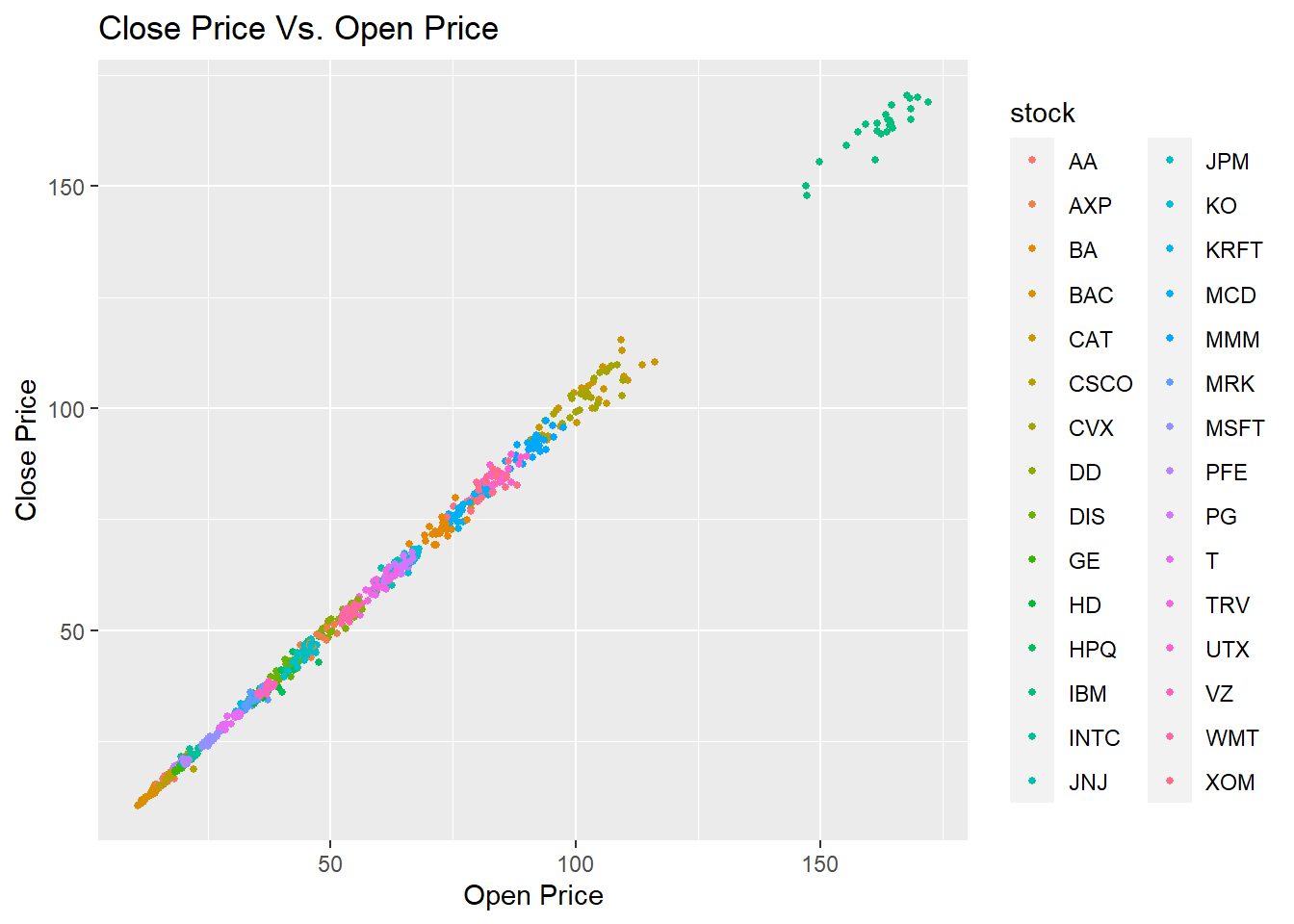``````library(ggplot2)
ggplot(data = input_data, aes(x=volume, y=close, color=stock)) + geom_point(size=1) + xlab("Volume") + ylab("Close Price") + ggtitle("Close Price Vs. Volume")``````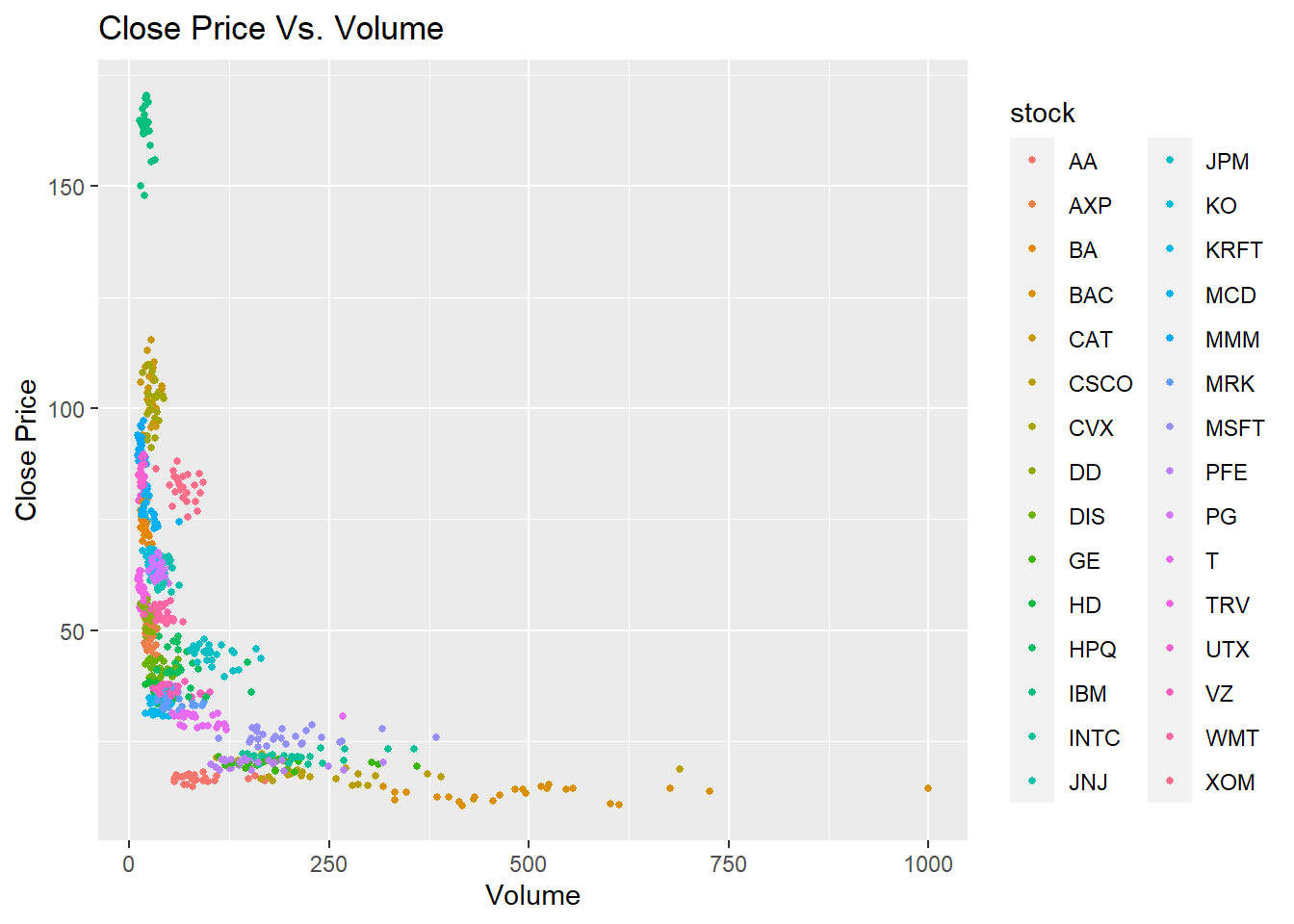## Line graphs

### Explanation:

These line graphs reveal the trends, seasonalities, and cycles of each stock.

### Observation:

Since this is stock data, a six-month of data would rarely capture the trends, seasonal patterns or cycles of these stocks. In order to see trends, seasonal patterns or cycles, at least a year worth of stock price data is neccessary.

``````library(ggplot2)
ggplot(data = input_data, aes(x=date, y=open, color=stock)) + geom_line(size=1) + xlab("Open Price") + ylab("Open Price") + ggtitle("Open Price Vs. Date")``````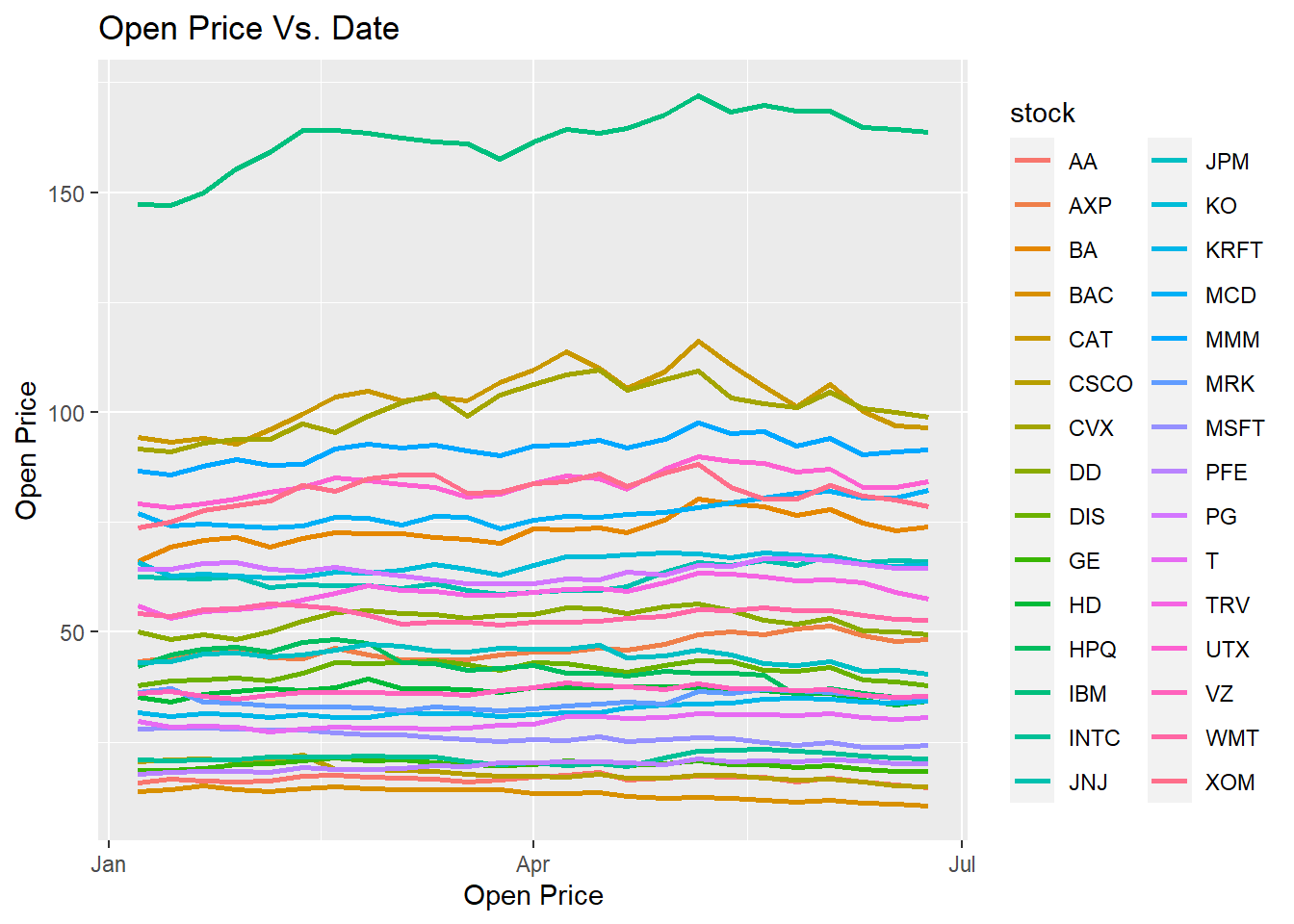``````library(ggplot2)
ggplot(data = input_data, aes(x=date, y=close, color=stock)) + geom_line(size=1) + xlab("Volume") + ylab("Close Price") + ggtitle("Close Price Vs. Date")``````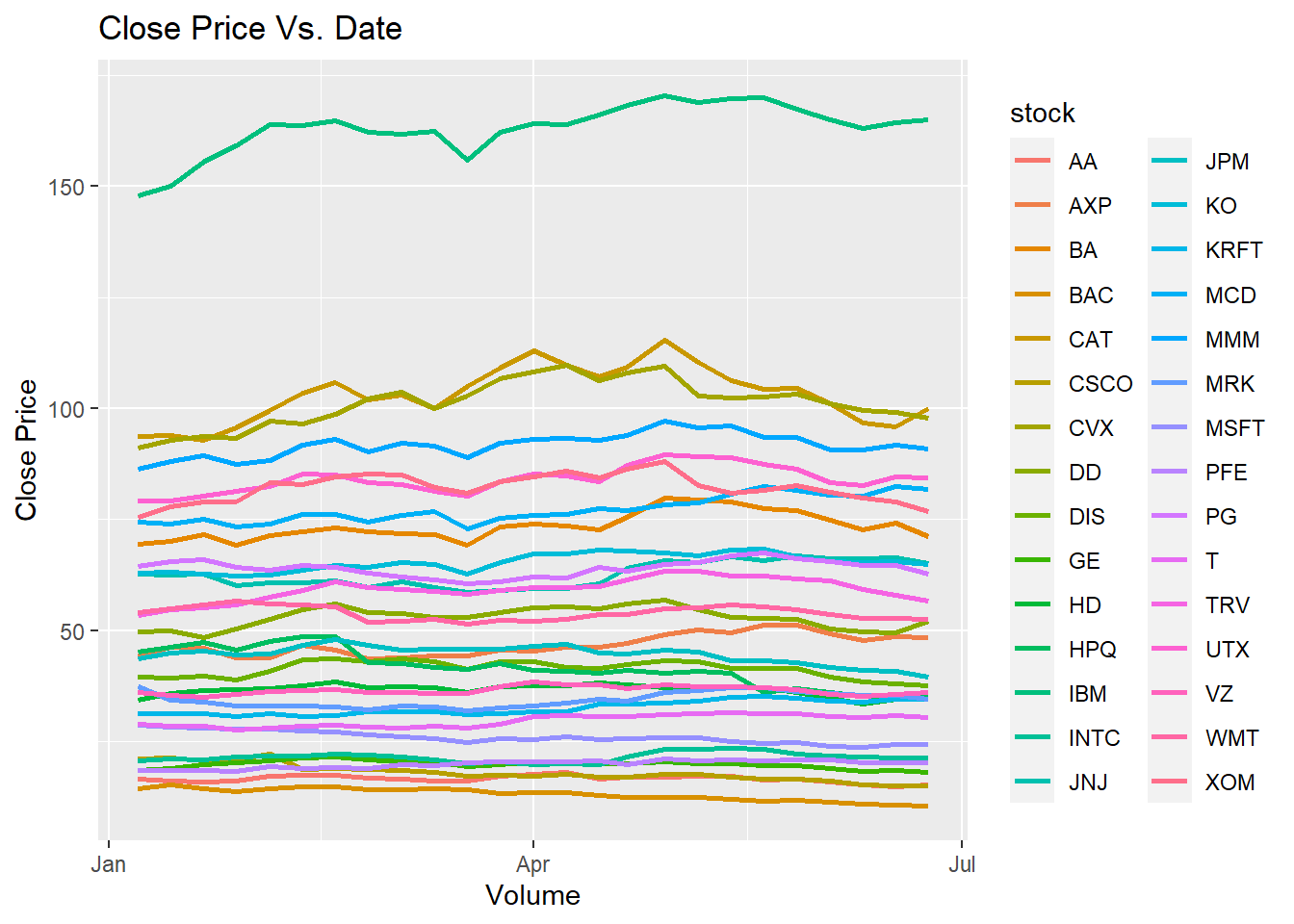``````library(ggplot2)
ggplot(data = input_data, aes(x=date, y=volume, color=stock)) + geom_line(size=1) + xlab("Volume") + ylab("Close Price") + ggtitle("Volume Vs. Date")``````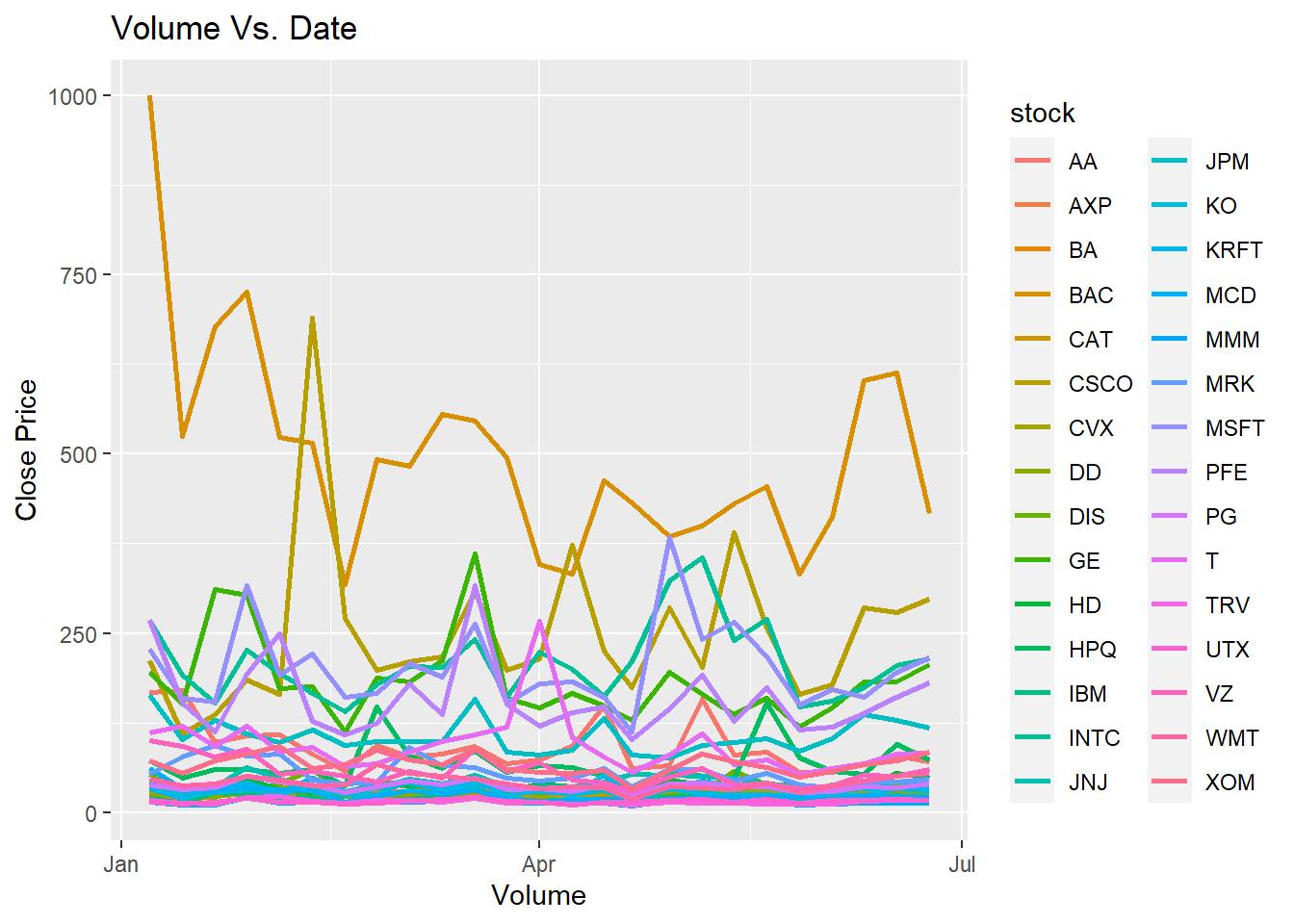## Quantile-quantile plot

### Explanation:

Plotting the Quantiles of actual data against the Quantiles of the normal distribution.

### Observation:

The Quantiles of some stock series do not seem to resemble the Quantiles of the normal distribution.

``````library(ggplot2)
ggplot(input_data, aes(sample = close, colour = factor(stock))) +  stat_qq() +  stat_qq_line()``````